Home System Programming Learn about Abstract Classes and Virtual Functions in C++

# Learn about Abstract Classes and Virtual Functions in C++

0
2633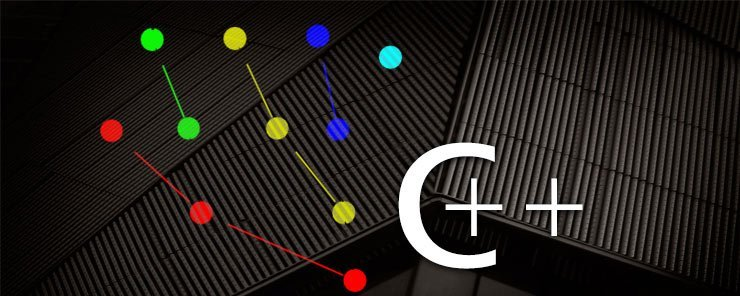In the previous article, we were  introduced to Inheritance. At the end of that article, I gave you a hint about Abstract Classes. I told you that an Abstract Class is a class that you can’t instantiate (create objects from), but are a sort of classes that serve as a base to derive other child classes from. For a class to be made abstract, it should have at least one pure virtual function.  Our focus today will be on Abstract Classes and Pure Virtual Functions. Happy reading.

Pure Virtual Functions
A pure virtual function is declared inside a base class, and “must” be implemented (overridden) by any non-abstract derived class.

Consider the following class definition:

```class A
{
protected:
int x;
public:
A(int ax)
{ x = ax; }
virtual void behave() = 0;
};
```

This is an abstract class.

Having the keyword virtual in the function declaration makes the function behave() like a virtual function. The trailing  = 0 part makes it pure virtual, and causes the class to be abstract.

Assume you don’t believe me, and want to make sure class A can never be instantiated (as I claim). OK, so simple; all you need to do is to try to create objects of class A:

```int main() {
A objecta(3);
return 0;
}
```

If you compile the above code, you will get an error like the following: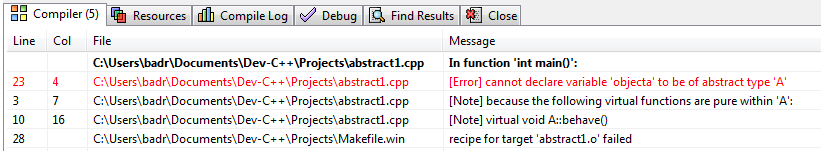The message shows clearly that you can’t declare variable (object) objecta to be of the type (class) A, as it is an abstract class.

Okay, let’s inherit that abstract class by a concrete (non-abstract) one:

```class B : public A
{
public:
B(int x) : A(x)
{ }
void behave()
{ cout << "\nx = " << x << "\nImplemented by derived class B\n"; }
};
```

Now, we need a main function to try this derived class:

```int main() {
B objectb(3);
objectb.behave();
return 0;
}
```

Executing this code should give the following output: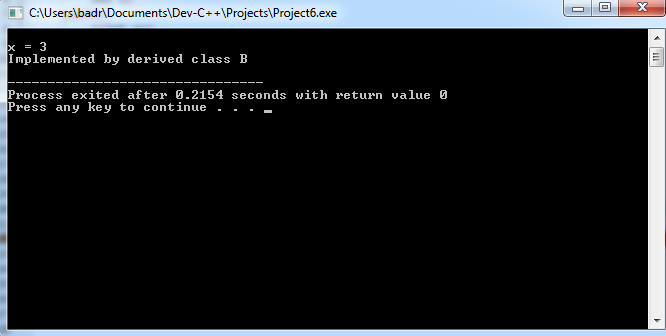Good job!

Public and Private Inheritance
One thing that we have passed by without clarification is the role of access specifier in the derived class definition line:

`class B : public A`

The word public here specifies that public member functions in the base class A are accessible to objects of class B. On the other hand, if we say:

`class B : private A`

This will prevent objects of class B from accessing public member functions in class A.

Inheriting from Multiple Classes
Unlike some other languages (Java) that don’t support multiple inheritance, C++ o allows a child class to inherit from more than one parent class.

Consider for example the circle class. A circle has a center (point) and at the same time is considered as a shape that has a perimeter and an area.

This means a circle actually inherits features from both point and shape classes. Let’s see how we can achieve this in C++ code.

```#include <iostream>
using namespace std;

class point
{
protected:
float x,y;
public:
point(float px, float py)
{ x = px; y = py; }
};

class shape				// Abstract Class
{
public:
virtual float perimeter() = 0;	//pure virtual function
virtual float area() = 0;		//another pure virtual function
};

/* The circle class will inherit from both point and shape */
class circle : public point, public shape
{
public:
circle(float x, float y, float rad) : point(x,y)
float perimeter()
{ return 2 * 3.14 * radius;	}
float area()
};
/*==========	The main() Function =========*/
int main() {
circle c(5,-1,4);
cout << "\nPerimeter: " << c.perimeter();
cout << "\nArea: " << c.area() << endl;
return 0;
}
```

How will this program execute? Let’s see: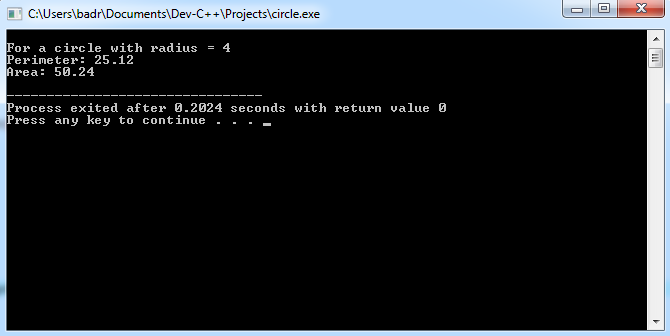Wonderful.

Aggregation (Composition)
Why to inherit a class (or more), if you could go ahead and use it (them) directly?!

In other words, class B inherits class A to make use of all features in class A. Couldn’t we do it by defining an object of class A inside class B?

Yes, we could. And this presents a simple alternative to multiple inheritance.
Consider the following code:

```#include <iostream.h>
#include <conio.h>
class ClassA
{
public:
void funcA()
{ cout << "Something done by Function A\n"; }
};
class ClassB
{
public:
void funcB()
{ cout << "Something done by Function B\n"; }
};
class ClassC
{
ClassA objA;
ClassB objB;
public:
void funcC()
{
objA.funcA();
objB.funcB();
cout << "Something else done by Function C\n";
}
};
void main()
{
clrscr();
ClassC c;
c.funcC();
getch();
}
```

Instead of inheriting ClassA and ClassB, the class ClassC has defined and used instances of both classes. When executed, the above program produces the following output: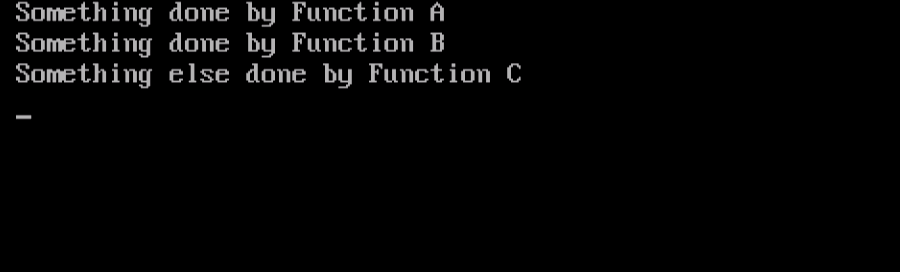Perfect!

Summary

• An abstract class is a class that you can’t instantiate. It serves as a base that derived classes can inherit.
• A class is considered abstract if it contains one or more pure virtual functions.
• A class can inherit from multiple classes the same way it inherits from one class.
• In Aggregation (composition), for a class B to utilize features in another class A, class B uses an object of class A, instead of inheriting class A.

In the next article, we will be talking about Pointers; the enigma of C++. An important part of the C++. See you there.